# Golang Tutorial – Learn Go Programming Language

• Difficulty Level : Hard
• Last Updated : 03 Feb, 2020

Golang or Go Programming Language is a statically-typed and procedural programming language having syntax similar to C language. It was developed in 2007 by Robert Griesemer, Rob Pike, and Ken Thompson at Google. But they launched it in 2009 as an open-source programming language. It provides a rich standard library, garbage collection, and dynamic-typing capability and also provides support for the environment adopting patterns alike to dynamic languages. The latest version of the Golang is 1.13.1 released on 3rd September 2019. Here, we are providing a complete tutorial of Golang with proper examples.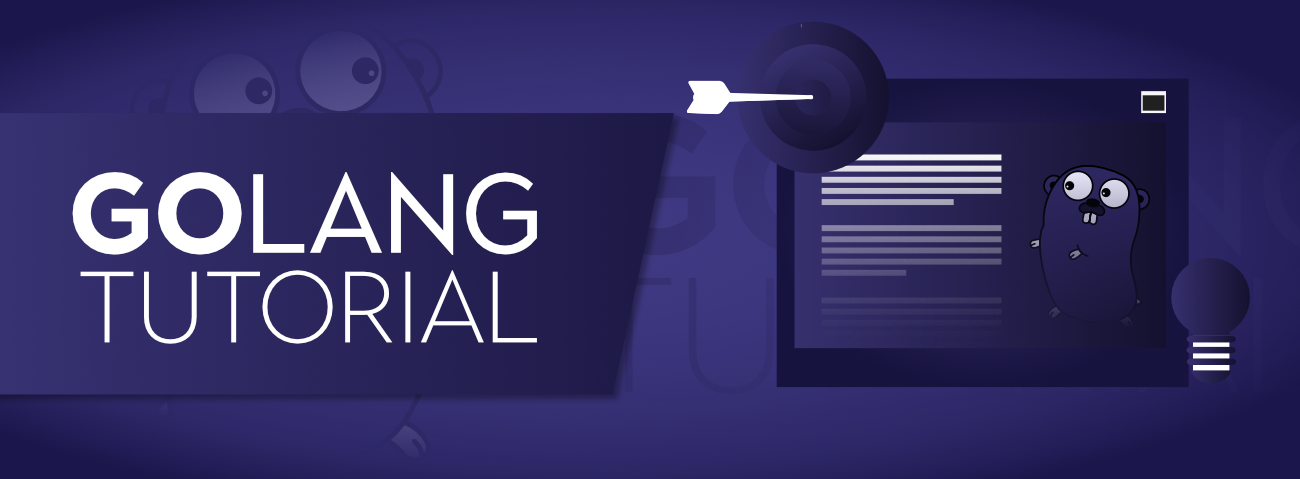Topics Covered:

### Why Golang?

The main purpose of designing Golang was to eliminate the problems of existing languages. So let us see the problems that we are facing with Python, Java, C/C++ programming languages:

• Python: It is easy to use but slow in compare to Golang.
• Java: It has very complex type system.
• C/C++: It has slow compilation time as well as complex type system.
• Also, all these languages were designed when multi-threading applications were rare, so not much effective to highly scalable, concurrent and parallel applications.
• Threading consumes 1MB whereas Goroutine consumes 2KB of memory, hence at the same time, we can have millions of goroutine triggered.

### Key Features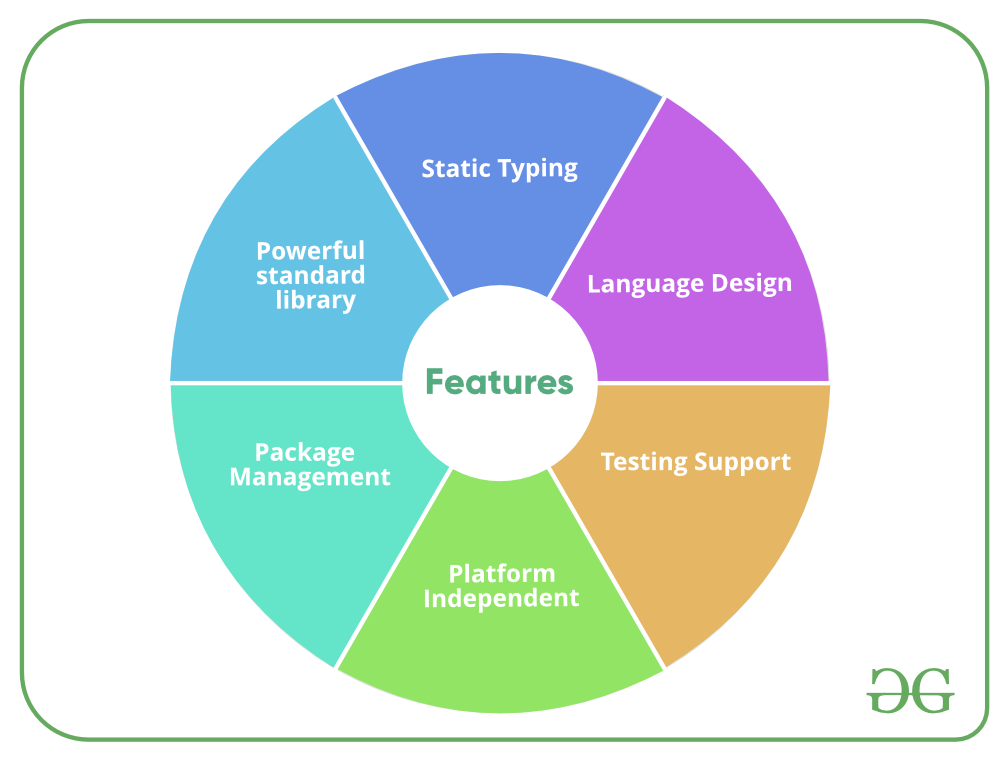Before we begin with the installation of Go, it is good to check if it might be already installed on your System. To check if your device is preinstalled with Golang or not, just go to the Command line(For Windows, search for cmd in the Run dialog( + R).

Now run the following command:

`go version`

If Golang is already installed, it will generate a message with all the details of the Golang’s version available, otherwise, if Golang is not installed then an error will arise stating Bad command or file name

Before starting with the installation process, you need to download it. For that, all versions of Go for Windows are available on golang.org.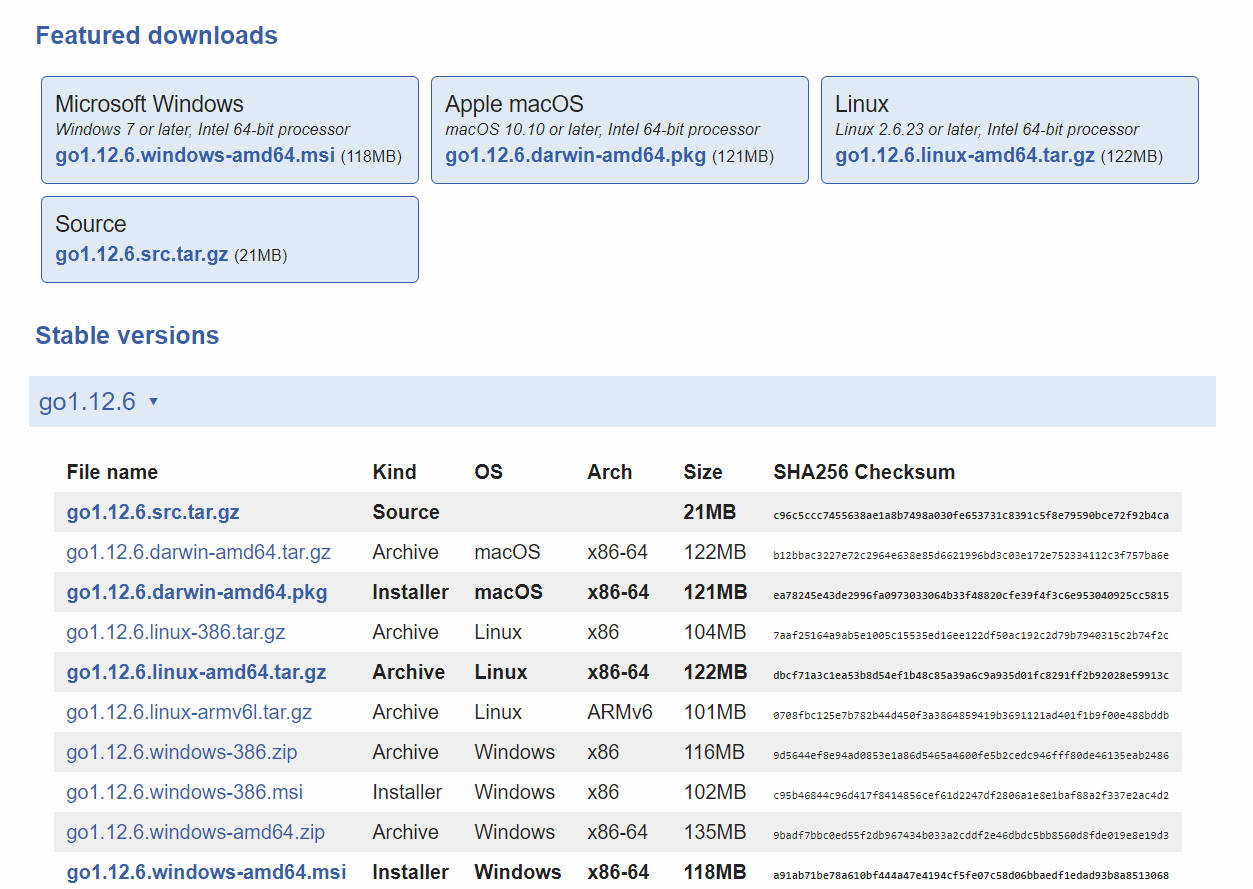Download the Golang according to your system architecture and follow the further instructions for the installation of Golang.

Step 1: After downloading, unzip the downloaded archive file. After unzipping you will get a folder named go in the current directory.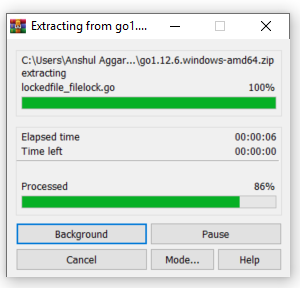Step 2: Now copy and paste the extracted folder wherever you want to install this. Here we are installing in C drive.

Step 3: Now set the environment variables. Right click on My PC and select Properties. Choose the Advanced System Settings from the left side and click on Environment Variables as shown in the below screenshots.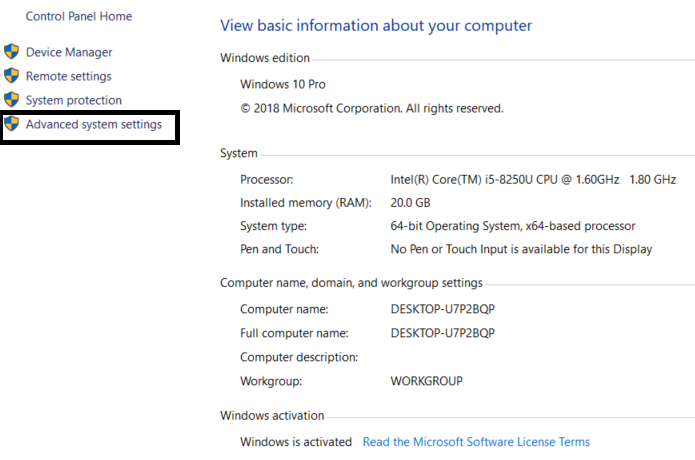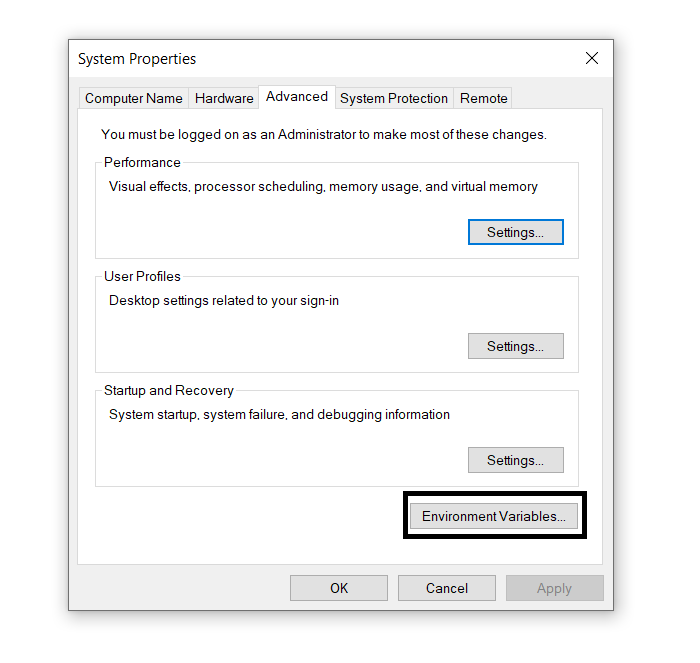Step 4: Click on Path from the system variables and then click Edit. Then Click New and then add the Path with bin directory where you have pasted the Go folder. Here we are editing the path C:\go\bin and click Ok as shown in the below screenshots.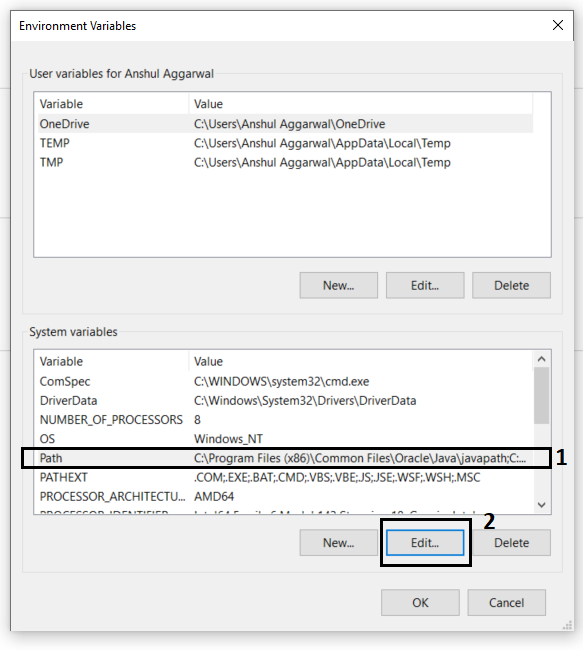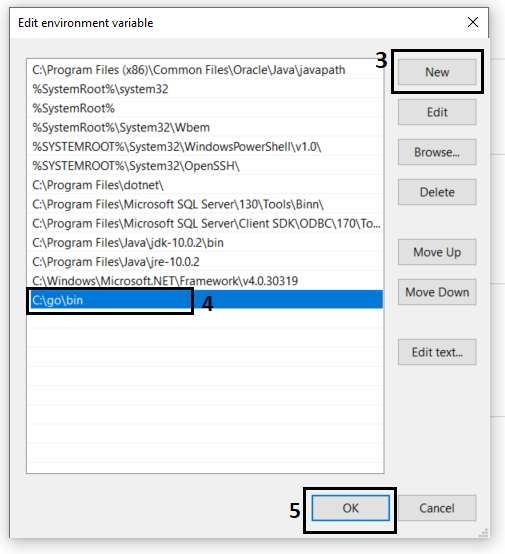Step 5: Now create a new user variable which tells Go command where Golang libraries are present. For that click on New on User Variables as shown in the below screenshots.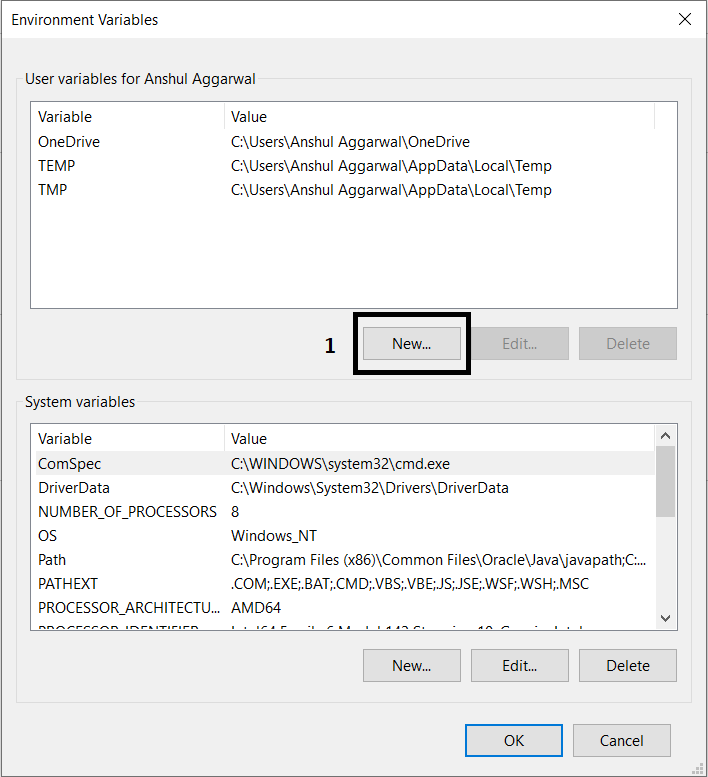Now fill the Variable name as GOROOT and Variable value is the path of your Golang folder. So here Variable Value is C:\go\. After Filling click OK.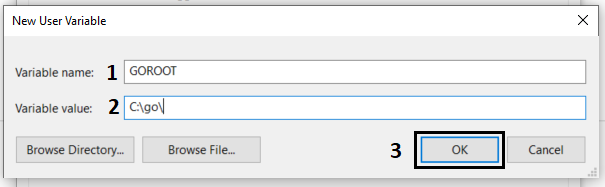After that Click Ok on Environment Variables and your setup is completed. Now Let’s check the Golang version by using the command `go version` on command prompt.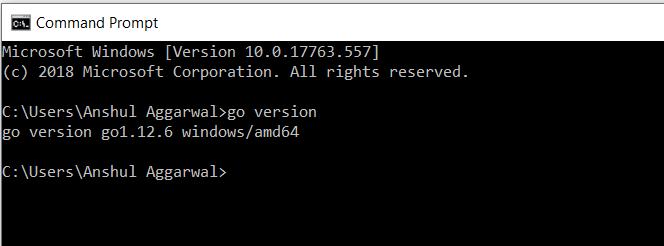After completing the installation process, any IDE or text editor can be used to write Golang Codes and Run them on the IDE or the Command prompt with the use of command:

`go run filename.go`

### Executing Hello World! Program

To run a Go program you need a Go compiler. In Go compiler, first you create a program and save your program with extension .go, for example, first.go.

 `// First Go program``package main`` ` `import ``"fmt"`` ` `// Main function``func main() {`` ` `    ``fmt.Println(``"!... Hello World ...!"``)``}`

Output:

`!... Hello World ...!`

Now we run this first.go file in the go compiler using the following command, i.e:

`\$ go run first.go`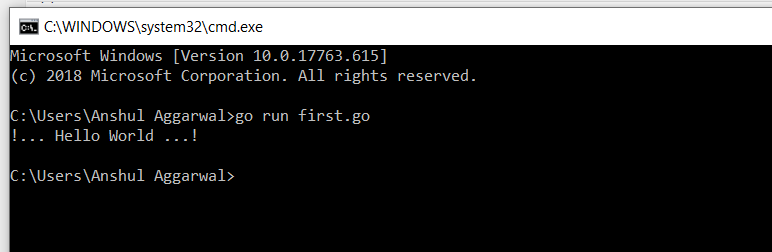For more details about the different terms used in this program, you can visit Hello World! in Golang

### Identifiers and Keywords

Identifiers are the user-defined name of the program components. In Go language, an identifier can be a variable name, function name, constant, statement labels, package name, or types.

Example:

```// Valid identifiers:
_geeks23
geeks
gek23sd
Geeks
geeKs
geeks_geeks

// Invalid identifiers:
212geeks
if
default
```

Keywords or Reserved words are the words in a language that are used for some internal process or represent some predefined actions. These words are therefore not allowed to use as an identifier. Doing this will result in a compile-time error. There are total 25 keywords present in the Go language as follows: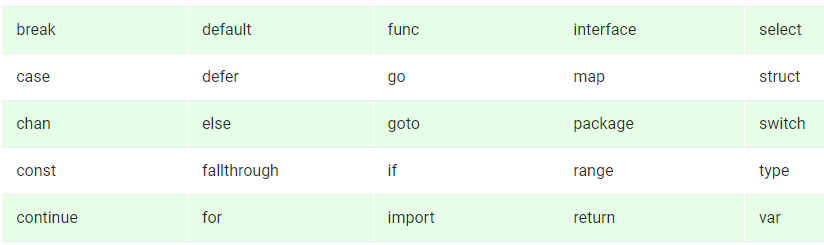Example:

 `// Go program to illustrate ``// the use of keywords`` ` `// Here package keyword is used to ``// include the main package``// in the program``package main`` ` `// import keyword is used to ``// import "fmt" in your package``import ``"fmt"`` ` `// func is used to``// create function``func main() {`` ` `    ``// Here, var keyword is used ``    ``// to create variables``    ``// Pname, Lname, and Cname ``    ``// are the valid identifiers``    ``var Pname = ``"GeeksforGeeks"` `    ``var Lname = ``"Go Language"` `    ``var Cname = ``"Keywords"``     ` `    ``fmt.Printf(``"Portal name: %s"``, Pname)``    ``fmt.Printf(``"\nLanguage name: %s"``, Lname)``    ``fmt.Printf(``"\nChapter name: %s"``, Cname)`` ` `}`

Output:

```Portal name: GeeksforGeeks
Language name: Go Language
Chapter name: Keywords
```

### Data Types

Data types specify the type of data that a valid Go variable can hold. In Go language, the type is divided into four categories which are as follows:

1. Basic type: Numbers, strings, and booleans come under this category.
2. Aggregate type: Array and structs come under this category.
3. Reference type: Pointers, slices, maps, functions, and channels come under this category.
4. Interface type

Here, we will discuss Basic Data Types in the Go language. The Basic Data Types are further categorized into three subcategories which are:

1. Numbers
2. Booleans
3. Strings

Numbers: In Go language, numbers are divided into three sub-categories that are:

• Integers: In Go language, both signed and unsigned integers are available in four different sizes as shown in the below table. The signed int is represented by int and the unsigned integer is represented by uint.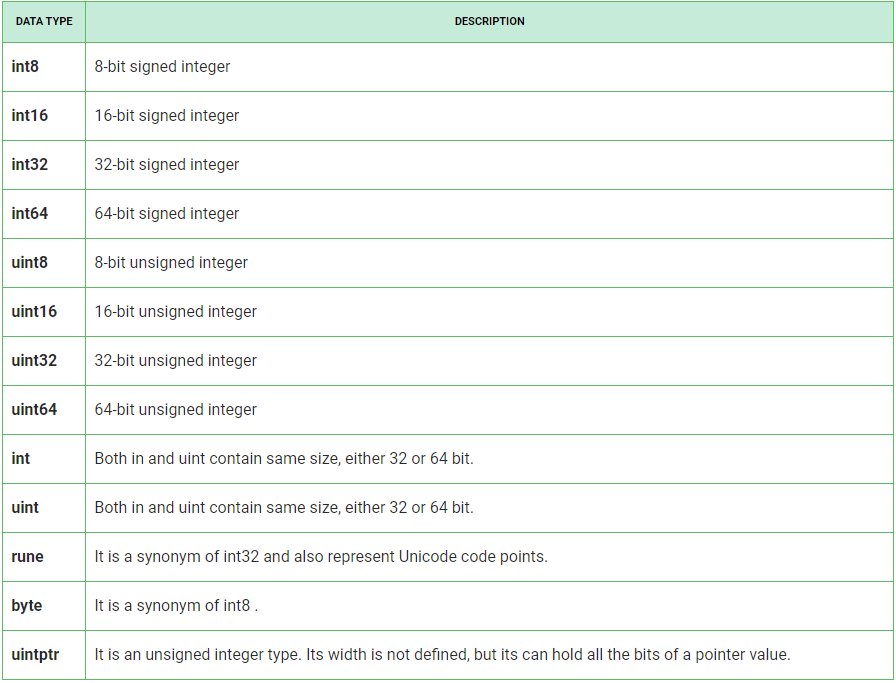• Floating-Point Numbers: In Go language, floating-point numbers are divided into two categories as shown in the below table: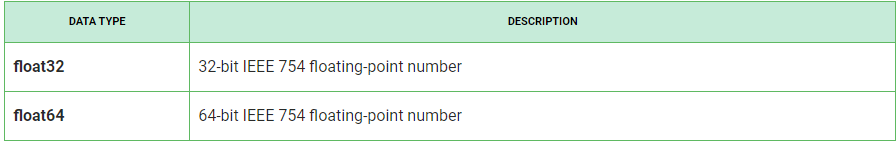• Complex Numbers: The complex numbers are divided into two parts are shown in the below table. float32 and float64 are also part of these complex numbers. The in-built function creates a complex number from its imaginary and real part and in-built imaginary and real function extract those parts.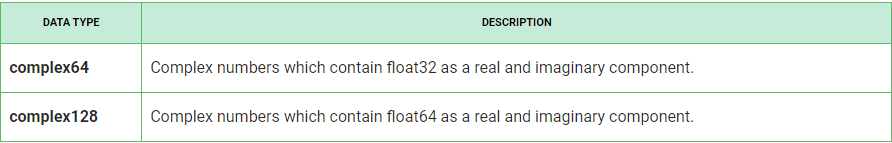• Example:

 `// Golang program to illustrate``// the use of integers, floating``// and complex numbers``package main`` ` `import ``"fmt"`` ` `func main() {`` ` `    ``// Using 8-bit unsigned int``    ``var X uint8 = 225``    ``fmt.Println(X+1, X)`` ` `    ``// Using 16-bit signed int``    ``var Y int16 = 32767``    ``fmt.Println(Y+2, Y-2)`` ` `    ``a := 20.45``    ``b := 34.89`` ` `    ``var m complex128 = complex(6, 2)``    ``var n complex64 = complex(9, 2)`` ` `    ``// Subtraction of two``    ``// floating-point number``    ``c := b - a`` ` `    ``// Display the result``    ``fmt.Printf(``"Result is: %f\n"``, c)`` ` `    ``// Display the type of c variable``    ``fmt.Printf(``"The type of c is : %T\n"``, c)`` ` `    ``fmt.Println(m)``    ``fmt.Println(n)`` ` `    ``// Display the type``    ``fmt.Printf(``"The type of m is %T and "``+``        ``"the type of n is %T"``, m, n)`` ` `}`

Output:

```226 225
-32767 32765
Result is: 14.440000
The type of c is : float64
(6+2i)
(9+2i)
The type of m is complex128 and the type of n is complex64
```

Booleans and Strings:

The boolean data type represents only one bit of information either true or false. The values of type boolean are not converted implicitly or explicitly to any other type.
The string data type represents a sequence of Unicode code points. Or in other words, we can say a string is a sequence of immutable bytes, means once a string is created you cannot change that string. A string may contain arbitrary data, including bytes with zero value in the human-readable form.

Example:

 `// Go program to illustrate``// the use of booleans and``// strings``package main`` ` `import ``"fmt"`` ` `func main() {`` ` `    ``// variables``    ``str1 := ``"GeeksforGeeks"``    ``str2 := ``"geeksForgeeks"``    ``result1 := str1 == str2`` ` `    ``// Display the result``    ``fmt.Println(result1)`` ` `    ``// Display the type of``    ``// result1``    ``fmt.Printf(``"The type of result1 is %T\n"``, result1)`` ` `    ``// str variable which stores strings``    ``str := ``"GeeksforGeeks"`` ` `    ``// Display the length of the string``    ``fmt.Printf(``"Length of the string is: %d"``, len(str))`` ` `    ``// Display the string``    ``fmt.Printf(``"\nString is: %s"``, str)`` ` `    ``// Display the type of str variable``    ``fmt.Printf(``"\nType of str is: %T"``, str)`` ` `}`

Output:

```false
The type of result1 is bool
Length of the string is: 13
String is: GeeksforGeeks
Type of str is: string
```

### Variables

A Variable is a placeholder of the information which can be changed at runtime. And variables allow to Retrieve and Manipulate the stored information.

Rules for Naming Variables:

• Variable names must begin with a letter or an underscore(_). And the names may contain the letters ‘a-z’ or ’A-Z’ or digits 0-9 as well as the character ‘_’.
```Geeks, geeks, _geeks23  // valid variable
123Geeks, 23geeks      // invalid variable
```
• A variable name should not start with a digit.
`234geeks  // illegal variable `
• The name of the variable is case sensitive.
`geeks and Geeks are two different variables`
• Keywords is not allowed to use as a variable name.
• There is no limit on the length of the name of the variable, but it is advisable to use an optimum length of 4 – 15 letters only.

There are two ways to declare a variable in Golang as follows:

1. Using var Keyword: In Go language, variables are created using var keyword of a particular type, connected with name and provide its initial value.

Syntax:

`var variable_name type = expression`

Example:

 `// Go program to illustrate ``// the use of var keyword``package main`` ` `import ``"fmt"`` ` `func main() {`` ` `// Variable declared and ``// initialized without the ``// explicit type``var myvariable1 = 20`` ` `// Display the value and the``// type of the variables``fmt.Printf(``"The value of myvariable1 is : %d\n"``,``                                  ``myvariable1)``                                     ` `fmt.Printf(``"The type of myvariable1 is : %T\n"``,``                                  ``myvariable1)``     ` `}`

Output:

```The value of myvariable1 is : 20
The type of myvariable1 is : int
```

To read more about the var keyword, you can refer to the article var keyword in Golang

2. Using short variable declaration: The local variables which are declared and initialize in the functions are declared by using short variable declaration.

Syntax:

`variable_name:= expression`

Note: Please don’t confuse in between := and = as := is a declaration and = is assignment.

Example:

 `// Go program to illustrate the``// short variable declaration ``package main``import ``"fmt"`` ` `func main() {`` ` `// Using short variable declaration``myvar1 := 39 `` ` `// Display the value and type of the variable``fmt.Printf(``"The value of myvar1 is : %d\n"``, myvar1)``fmt.Printf(``"The type of myvar1 is : %T\n"``, myvar1)`` ` `}`

Output:

```The value of myvar1 is : 39
The type of myvar1 is : int
```

To read more about the short variable declaration keyword, you can refer to the article Short Variable Declaration Operator(:=) in Golang

### Constants

As the name constants suggest means fixed, in programming languages also it is same i.e., once the value of constant is defined it cannot be modified further. There can be any basic data types of constant like an integer constant, a floating constant, a character constant, or a string literal.

How to declare?
Constant are declared like variables but in using a const keyword as a prefix to declare constant with a specific type. It cannot be declare using := syntax.

Example:

 `// Golang program to illustrate ``// the constants``package main`` ` `import ``"fmt"`` ` `const` `PI = 3.14`` ` `func main() {``    ``const` `GFG = ``"GeeksforGeeks"``    ``fmt.Println(``"Hello"``, GFG)`` ` `    ``fmt.Println(``"Happy"``, PI, ``"Day"``)`` ` `    ``const` `Correct = ``true``    ``fmt.Println(``"Go rules?"``, Correct)``}`

Output:

```Hello GeeksforGeeks
Happy 3.14 Day
Go rules? true
```

To read more about Constants in Golang, you can refer to the article Constants in Golang.

### Operators

Operators are the foundation of any programming language. Thus the functionality of the Go language is incomplete without the use of operators. Operators allow us to perform different kinds of operations on operands. In Go language, operators Can be categorized based upon their different functionality:

Example:

 `// Golang program to illustrate``// the use of operators``package main`` ` `import ``"fmt"`` ` `func main() {``    ``p := 23``    ``q := 60`` ` `    ``// Arithmetic Operator - Addition``    ``result1 := p + q``    ``fmt.Printf(``"Result of p + q = %d\n"``, result1)`` ` `    ``// Relational Operators - ‘=='(Equal To)``    ``result2 := p == q``    ``fmt.Println(result2)`` ` `    ``// Relational Operators - ‘!='(Not Equal To)``    ``result3 := p != q``    ``fmt.Println(result3)`` ` `    ``// Logical Operators``    ``if` `p != q && p <= q {``        ``fmt.Println(``"True"``)``    ``}`` ` `    ``if` `p != q || p <= q {``        ``fmt.Println(``"True"``)``    ``}`` ` `    ``if` `!(p == q) {``        ``fmt.Println(``"True"``)``    ``}`` ` `    ``// Bitwise Operators - & (bitwise AND)``    ``result4 := p & q``    ``fmt.Printf(``"Result of p & q = %d\n"``, result4)`` ` `    ``// Assignment Operators - “=”(Simple Assignment)``    ``p = q``    ``fmt.Println(p)`` ` `}`

Output:

```Result of p + q = 83
false
true
True
True
True
Result of p & q = 20
60
```

### Control Flow

Decision Making Statements

Decision Making in programming is similar to decision making in real life. A piece of code is executed when the given condition is fulfilled. Sometimes these are also termed as the Control flow statements. A programming language uses control statements to control the flow of execution of the program based on certain conditions. These are used to cause the flow of execution to advance and branch based on changes to the state of a program.

• if : It is used to decide whether a certain statement or block of statements will be executed or not i.e if a certain condition is true then a block of statement is executed otherwise not.

Syntax:

```if(condition) {

// Statements to execute if
// condition is true
}
```

Flow Chart: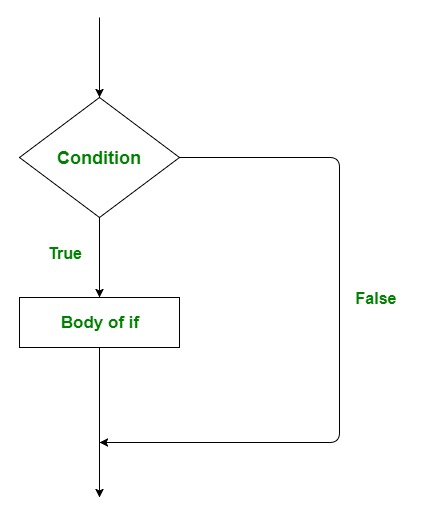• if-else : if we want to do something else if the condition is false. Here comes the else statement. We can use the else statement with if statement to execute a block of code when the condition is false.

Syntax:

```
if (condition) {

// Executes this block if
// condition is true
} else {

// Executes this block if
// condition is false
}
```

Flow Chart: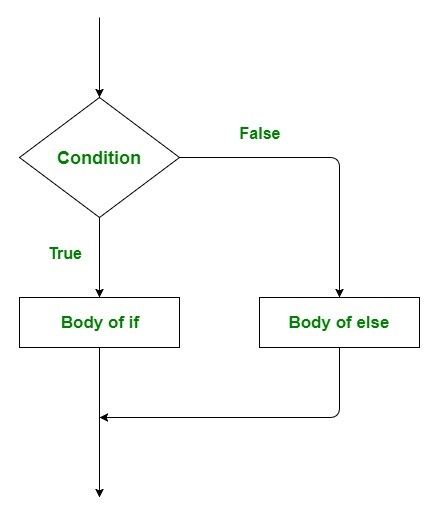• Nested if : Nested if statements mean an if statement inside an if statement. Yes, Golang allows us to nest if statements within if statements. i.e, we can place an if statement inside another if statement.

Syntax:

```if (condition1) {

// Executes when condition1 is true

if (condition2) {

// Executes when condition2 is true
}
}
```

Flow Chart: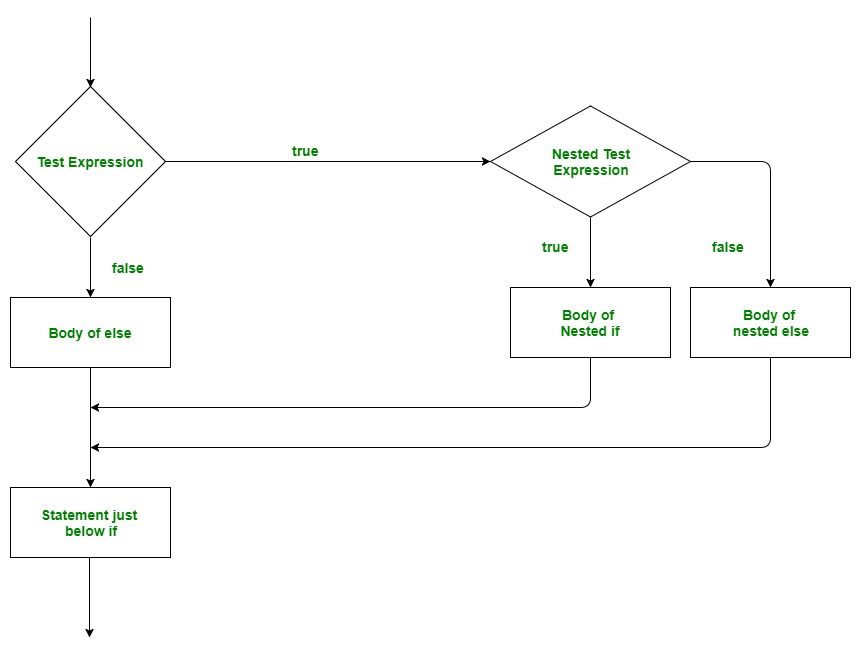• if-else-if Ladder : Here, a user can decide among multiple options. The if statements are executed from the top down. As soon as one of the conditions controlling the if is true, the statement associated with that if is executed, and the rest of the ladder is bypassed. If none of the conditions is true, then the final else statement will be executed.

Important Points:

• if statement can have zero or one else’s and it must come after any else if’s.
• if statement can have zero to many else if’s and it must come before the else.
• None of the remaining else if’s or else’s will be tested if an else if succeeds,

Syntax:

```if(condition_1) {

// this block will execute
// when condition_1 is true

} else if(condition_2) {

// this block will execute
// when condition2 is true
}
.
.
. else {

// this block will execute when none
// of the condition is true
}
```

Flow Chart: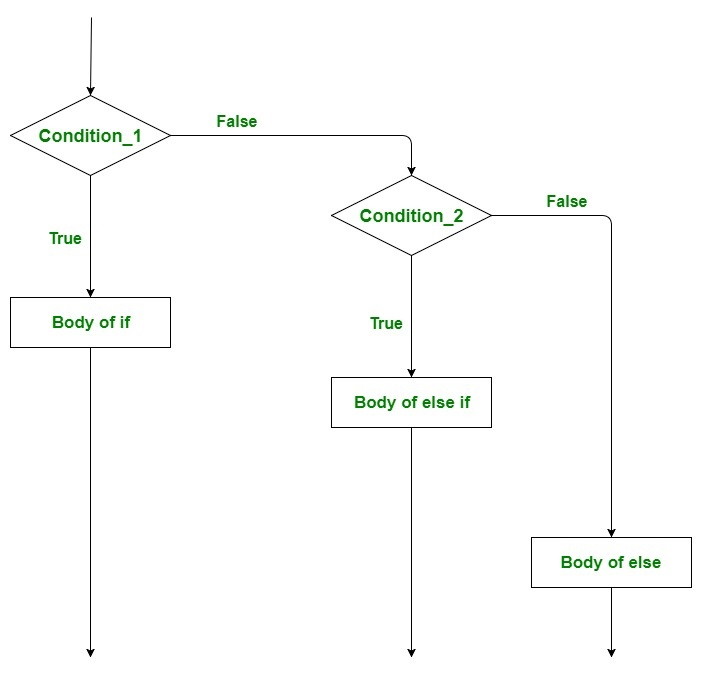Example 1: To demonstrate the if and if-else statement

 `// Golang program to illustrate``// the use of if and if-else``// statement``package main`` ` `import ``"fmt"`` ` `func main() {`` ` `    ``// taking local variables``    ``var a ``int` `= 100``    ``var b ``int` `= 175`` ` `    ``// using if statement for``    ``// checking the condition``    ``if` `a%2 == 0 {`` ` `        ``// print the following if``        ``// condition evaluates to true``        ``fmt.Printf(``"Even Number\n"``)`` ` `    ``}`` ` `    ``if` `b%2 == 0 {`` ` `        ``fmt.Printf(``"Even Number"``)`` ` `    ``} ``else` `{`` ` `        ``fmt.Printf(``"Odd Number"``)``    ``}``}`

Output:

```Even Number
Odd Number
```

Example 2: To demonstrate the Nested-if and if-else-if ladder statement

 `// Golang program to illustrate``// the use of nested if and``// if-else-if ladder statement``// statement``package main`` ` `import ``"fmt"`` ` `func main() {`` ` `    ``// taking two local variable``    ``var v1 ``int` `= 400``    ``var v2 ``int` `= 700`` ` `    ``// ----- Nested if Statement -------`` ` `    ``// using if statement``    ``if` `v1 == 400 {`` ` `        ``// if condition is true then``        ``// check the following``        ``if` `v2 == 700 {`` ` `            ``// if condition is true``            ``// then display the following``            ``fmt.Printf(``"Value of v1 is 400 and v2 is 700\n"``)``        ``}``    ``}`` ` `    ``// ----------- if-else-if ladder``     ` `    ``// checking the condition``    ``if` `v1 == 100 {`` ` `        ``// if condition is true then``        ``// display the following */``        ``fmt.Printf(``"Value of v1 is 100\n"``)`` ` `    ``} ``else` `if` `v1 == 200 {`` ` `        ``fmt.Printf(``"Value of a is 20\n"``)`` ` `    ``} ``else` `if` `v1 == 300 {`` ` `        ``fmt.Printf(``"Value of a is 300\n"``)`` ` `    ``} ``else` `{`` ` `        ``// if none of the conditions is true``        ``fmt.Printf(``"None of the values is matching\n"``)``    ``}`` ` `}`

Output:

```Value of v1 is 400 and v2 is 700
None of the values is matching
```

for loop

Go language contains only a single loop that is for-loop. A for loop is a repetition control structure that allows us to write a loop that is executed a specific number of times. A simple for loop is similar that we use in other programming languages like C, C++, Java, C#, etc.
Syntax:

```for initialization; condition; post{
// statements....
}
```

Here,

• The initialization statement is optional and executes before for loop starts. The initialization statement is always in a simple statement like variable declarations, increment or assignment statements, or function calls.
• The condition statement holds a boolean expression, which is evaluated at the starting of each iteration of the loop. If the value of the conditional statement is true, then the loop executes.
• The post statement is executed after the body of the for-loop. After the post statement, the condition statement evaluates again if the value of the conditional statement is false, then the loop ends.

Example:

 `// Go program to illustrate the  ``// use of simple for loop ``package main`` ` `import ``"fmt"`` ` `// Main function``func main() {``     ` `    ``// for loop ``    ``// This loop starts when i = 0 ``    ``// executes till i<4 condition is true``    ``// post statement is i++``    ``for` `i := 0; i < 4; i++{``      ``fmt.Printf(``"GeeksforGeeks\n"``)  ``    ``}``   ` `}`

Output:

```GeeksforGeeks
GeeksforGeeks
GeeksforGeeks
GeeksforGeeks
```

Note: This for loop can be used as Infinite loop and while loop. To read more about for loop you can refer to the article Loops in Golang.

### Loop Control Statements

Loop control statements in the Go language are used to change the execution of the program. When the execution of the given loop left its scope, then the objects that are created within the scope are also demolished. The Go language supports 3 types of loop control statements:

1. break
2. goto
3. continue

#### break Statement

The break statement is used to terminate the loop or statement in which it presents. After that, the control will pass to the statements that present after the break statement, if available. If the break statement present in the nested loop, then it terminates only those loops which contains break statement.

Flow Chart: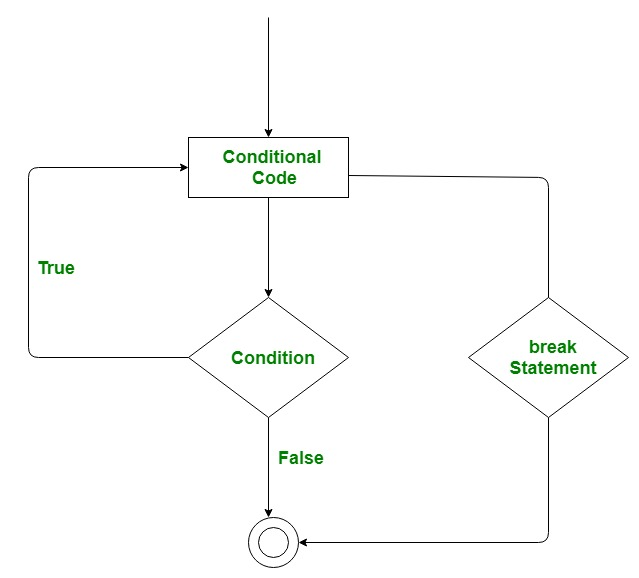Example:

 `// Go program to illustrate ``// the use of break statement``package main`` ` `import ``"fmt"`` ` `// Main function``func main() {``   ``for` `i:=0; i<5; i++{``        ` `   ``fmt.Println(i)``    ` `   ``// For loop breaks when the value of i = 3``   ``if` `i == 3{``         ``break``;``  ``}``   ``}`` ` `    ` `}`

Output:

```0
1
2
3
```

#### goto Statement

This statement is used to transfer control to the labeled statement in the program. The label is the valid identifier and placed just before the statement from where the control is transferred. Generally, goto statement is not used by the programmers because it is difficult to trace the control flow of the program.

Flow Chart: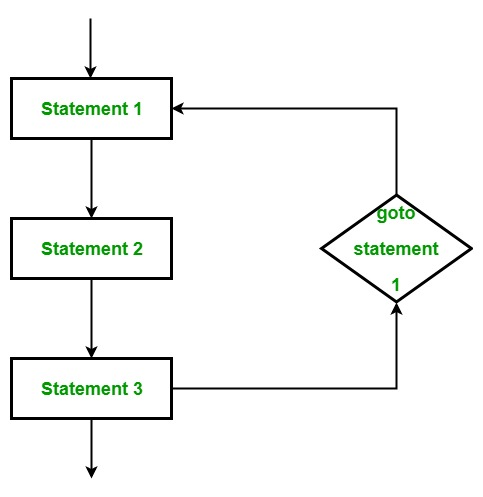Example:

 `// Go program to illustrate ``// the use of goto statement``package main`` ` `import ``"fmt"`` ` `func main() {``   ``var x ``int` `= 0``    ` `   ``// for loop work as a while loop``  ``Lable1: ``for` `x < 8 {``      ``if` `x == 5 {``          ` `         ``// using goto statement``         ``x = x + 1;``         ``goto` `Lable1``      ``}``      ``fmt.Printf(``"value is: %d\n"``, x);``      ``x++;     ``   ``}  ``}`

Output:

```value is: 0
value is: 1
value is: 2
value is: 3
value is: 4
value is: 6
value is: 7
```

#### continue Statement

This statement is used to skip over the execution part of the loop on a certain condition. After that, it transfers the control to the beginning of the loop. It skips its following statements and continues with the next iteration of the loop.

Flow Chart: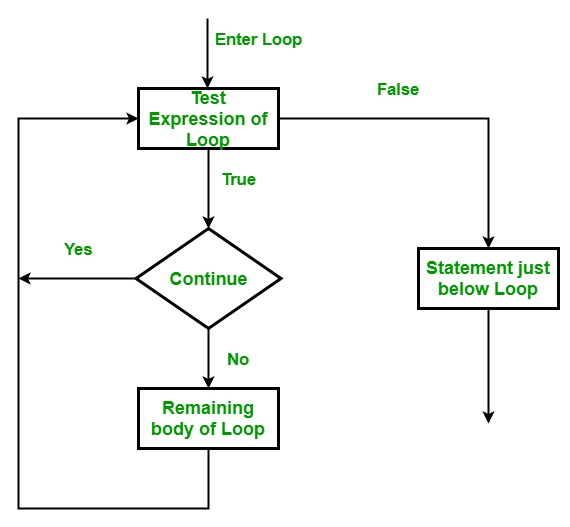Example:

 `// Go program to illustrate ``// the use of continue statement``package main`` ` `import ``"fmt"`` ` `func main() {``   ``var x ``int` `= 0``    ` `   ``// for loop work as a while loop``   ``for` `x < 8 {``      ``if` `x == 5 {``          ` `         ``// skip two iterations``         ``x = x + 2;``         ``continue``;``      ``}``      ``fmt.Printf(``"value is: %d\n"``, x);``      ``x++;     ``   ``}  ``}`

Output:

```value is: 0
value is: 1
value is: 2
value is: 3
value is: 4
value is: 7
```

### Switch Statement

A switch statement is a multiway branch statement. It provides an efficient way to transfer the execution to different parts of a code based on the value(also called case) of the expression. Even we can add multiple values in the case statement by using a comma.

Syntax:

```switch expression {
case value_1:
statement......1
case value_2:
statement......2
case value_n:
statement......n
default:
statement......default
}
```

Example:

 `// Go program to illustrate the  ``// concept of switch statement ``package main ``   ` `import ``"fmt"``   ` `func main() { ``    ``var value string = ``"five"``       ` `    ``// Switch statement without default statement ``    ``// Multiple values in case statement ``   ``switch` `value { ``       ``case` `"one"``: ``       ``fmt.Println(``"C#"``) ``       ``case` `"two"``, ``"three"``: ``       ``fmt.Println(``"Go"``) ``       ``case` `"four"``, ``"five"``, ``"six"``: ``       ``fmt.Println(``"Golang"``) ``   ``}   ``} `

Output:

```Golang
```

To read more about switch statement you can refer to the article Switch Statement in Golang

### Arrays

An array is a fixed-length sequence that is used to store homogeneous elements in the memory. Due to their fixed length array are not much popular like Slice in Go language. In an array, you are allowed to store zero or more than zero elements in it. The elements of the array are indexed by using the [] index operator with their zero-based position, means the index of the first element is array and the index of the last element is array[len(array)-1].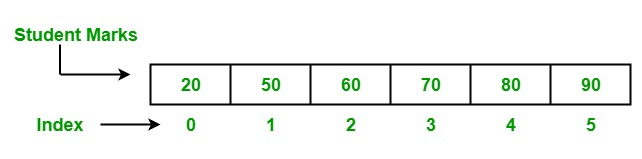There are two ways to create an array in Golang as follows:

1. Using var keyword: In Go language, an array is created using the var keyword of a particular type with name, size, and elements.

Syntax:

```Var array_name[length]Type
or
var array_name[length]Typle{item1, item2, item3, ...itemN}
```

In Go language, arrays are mutable, so that you can use array[index] syntax to the left-hand side of the assignment to set the elements of the array at the given index.

`Var array_name[index] = element`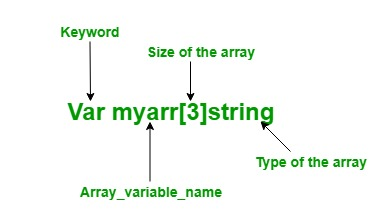2. Using shorthand declaration: In Go language, arrays can also declare using the shorthand declaration. It is more flexible than the above declaration.

Syntax:

`array_name:= [length]Type{item1, item2, item3, ...itemN}`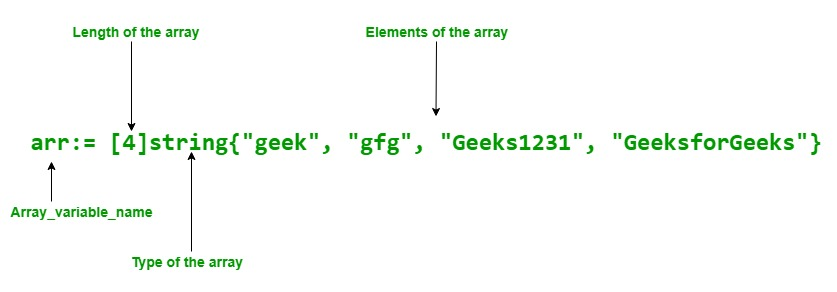Example:

 `// Golang program to illustrate the arrays``package main`` ` `import ``"fmt"`` ` `func main() {`` ` `    ``// Creating an array of string type``    ``// Using var keyword``    ``var myarr string`` ` `    ``// Elements are assigned using index``    ``myarr = ``"GFG"``    ``myarr = ``"GeeksforGeeks"`` ` `    ``// Accessing the elements of the array``    ``// Using index value``    ``fmt.Println(``"Elements of Array:"``)``    ``fmt.Println(``"Element 1: "``, myarr)``    ``fmt.Println(``"Element 2: "``, myarr)`` ` `    ``// Shorthand declaration of array``    ``arr := string{``"geek"``, ``"gfg"``, ``"Geeks1231"``, ``"GeeksforGeeks"``}`` ` `    ``// Accessing the elements of``    ``// the array Using for loop``    ``fmt.Println(``"\nElements of the array:"``)`` ` `    ``for` `i := 0; i < 3; i++ {``        ``fmt.Println(arr[i])`` ` `    ``}`` ` `}`

Output:

```Elements of Array:
Element 1:  GFG
Element 2:  GeeksforGeeks

Elements of the array:
geek
gfg
Geeks1231
```

To read more about arrays, you can refer to the article Arrays in Golang

### Slices

Slice is more powerful, flexible, convenient than an array, and is a lightweight data structure. Slice is a variable-length sequence which stores elements of a similar type, you are not allowed to store different type of elements in the same slice. It is just like an array having an index value and length, but the size of the slice is resized they are not in fixed-size just like an array. Internally, slice and an array are connected, a slice is a reference to an underlying array. It is allowed to store duplicate elements in the slice. The first index position in a slice is always 0 and the last one will be (length of slice – 1).

Syntax for Declaration:

```[]T
or
[]T{}
or
[]T{value1, value2, value3, ...value n}
```

Here, T is the type of the elements. For example:

`var my_slice[]int`

Pointer, Length, and Capacity are the main three components of the slice.

Example:

 `// Golang program to illustrate``// the working of the slice``package main`` ` `import ``"fmt"`` ` `func main() {`` ` `    ``// Creating an array``    ``arr := string{``"This"``, ``"is"``, ``"the"``, ``"tutorial"``,``                         ``"of"``, ``"Go"``, ``"language"``}`` ` `    ``// Display array``    ``fmt.Println(``"Array:"``, arr)`` ` `    ``// Creating a slice``    ``myslice := arr[1:6]`` ` `    ``// Display slice``    ``fmt.Println(``"Slice:"``, myslice)`` ` `    ``// Display length of the slice``    ``fmt.Printf(``"Length of the slice: %d"``, len(myslice))`` ` `    ``// Display the capacity of the slice``    ``fmt.Printf(``"\nCapacity of the slice: %d"``, cap(myslice))``}`

Output:

```Array: [This is the tutorial of Go language]
Slice: [is the tutorial of Go]
Length of the slice: 5
Capacity of the slice: 6
```

Explanation: In the above example, we create a slice from the given array. Here the pointer of the slice pointed to index 1 because the lower bound of the slice is set to one so it starts accessing elements from index 1. The length of the slice is 5, which means the total number of elements present in the slice is 5 and the capacity of the slice 6 means it can store a maximum of 6 elements in it.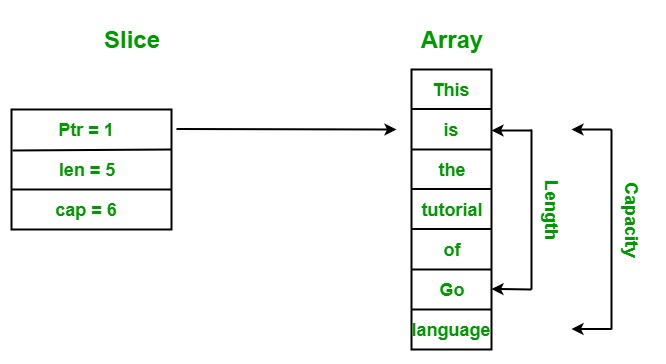To read more about slices you can refer to the article Slices in Golang

### Functions

Functions are generally the block of codes or statements in a program that gives the user the ability to reuse the same code which ultimately saves the excessive use of memory, acts as a time saver and more importantly, provides better readability of the code. So basically, a function is a collection of statements that perform some specific task and return the result to the caller. A function can also perform some specific task without returning anything.

Syntax:

```func function_name(Parameter-list)(Return_type){
// function body.....
}
```

You can return multiple values from the function. Also, the parameters and returns types are optional.

Example:

 `// Go program to illustrate the``// use of function``package main``import ``"fmt"`` ` `// area() is used to find the ``// area of the rectangle``// area() function two parameters,``// i.e, length and width``func area(length, width ``int``)``int``{``     ` `    ``Ar := length* width``    ``return` `Ar``}`` ` `// Main function``func main() {``   ` `   ``// Display the area of the rectangle``   ``// with method calling``   ``fmt.Printf(``"Area of rectangle is : %d"``, area(12, 10))``}`

Output:

`Area of rectangle is : 120`

To read more about the Functions you can refer to the article Functions in Golang.

### Structures

It is a user-defined type that allows to group/combine items of possibly different types into a single type. It can be termed as a lightweight class that does not support inheritance but supports composition.

First, you need to declare a structure type using the below syntax:

```type struct_name struct {
variable_1 type_of_variable_1
variable_2 type_of_variable_2
variable_n type_of_variable_3
}
```

Second, you have to create variables of that type to store values.

```var variable_name struct_name
```

Example:

 `// Golang program to show how to``// declare and define the struct`` ` `package main`` ` `import ``"fmt"`` ` `// Defining a struct type``type Address ``struct` `{``    ``Name    string``    ``city    string``    ``Pincode ``int``}`` ` `func main() {`` ` `    ``// Declaring a variable of a `struct` type``    ``// All the struct fields are initialized ``    ``// with their zero value``    ``var a Address ``    ``fmt.Println(a)`` ` `    ``// Declaring and initializing a``    ``// struct using a struct literal``    ``a1 := Address{``"Akshay"``, ``"Dehradun"``, 3623572}`` ` `    ``fmt.Println(``"Address1: "``, a1)`` ` `    ``// Naming fields while ``    ``// initializing a struct``    ``a2 := Address{Name: ``"Anikaa"``, city: ``"Ballia"``,``                                 ``Pincode: 277001}`` ` `    ``fmt.Println(``"Address2: "``, a2)`` ` `    ``// Uninitialized fields are set to``    ``// their corresponding zero-value``    ``a3 := Address{Name: ``"Delhi"``}``    ``fmt.Println(``"Address3: "``, a3)``}`

Output:

```{  0}
Address2:  {Anikaa Ballia 277001}
```

To access individual fields of a struct you have to use dot (.) operator. To read more about structures you can refer to the article Structure in Golang.

### Packages

The purpose of a package is to design and maintain a large number of programs by grouping related features together into single units so that they can be easy to maintain and understand and independent of the other package programs. In Go language, every package is defined with a different name and that name is close to their functionality like “strings” package and it contains methods and functions that only related to strings.

Example:

 `// Go program to illustrate the``// concept of packages``// Package declaration``package main`` ` `// Importing multiple packages``import (``    ``"bytes"``    ``"fmt"``    ``"sort"``)`` ` `func main() {`` ` `    ``// Creating and initializing slice``    ``// Using shorthand declaration``    ``slice_1 := []byte{``'*'``, ``'G'``, ``'e'``, ``'e'``, ``'k'``, ``'s'``, ``'f'``,``        ``'o'``, ``'r'``, ``'G'``, ``'e'``, ``'e'``, ``'k'``, ``'s'``, ``'^'``, ``'^'``}``    ``slice_2 := []string{``"Gee"``, ``"ks"``, ``"for"``, ``"Gee"``, ``"ks"``}`` ` `    ``// Displaying slices``    ``fmt.Println(``"Original Slice:"``)``    ``fmt.Printf(``"Slice 1 : %s"``, slice_1)``    ``fmt.Println(``"\nSlice 2: "``, slice_2)`` ` `    ``// Trimming specified leading``    ``// and trailing Unicode points``    ``// from the given slice of bytes``    ``// Using Trim function``    ``res := bytes.Trim(slice_1, ``"*^"``)``    ``fmt.Printf(``"\nNew Slice : %s"``, res)`` ` `    ``// Sorting slice 2``    ``// Using Strings function``    ``sort.Strings(slice_2)``    ``fmt.Println(``"\nSorted slice:"``, slice_2)``}`

Output:

```Original Slice:
Slice 1 : *GeeksforGeeks^^
Slice 2:  [Gee ks for Gee ks]

New Slice : GeeksforGeeks
Sorted slice: [Gee Gee for ks ks]
```

### Defer

It is keyword which is delay the execution of the function or method or an anonymous method until the nearby functions returns. Or in other words, defer function or method call arguments evaluate instantly, but they execute until the nearby functions returns.

Example:

 `// Go program to illustrate the``// concept of the defer statement``package main`` ` `import ``"fmt"`` ` `// Functions``func mul(a1, a2 ``int``) ``int` `{`` ` `    ``res := a1 * a2``    ``fmt.Println(``"Result: "``, res)``    ``return` `0``}`` ` `func show() {``    ``fmt.Println(``"Hello!, GeeksforGeeks"``)``}`` ` `// Main function``func main() {`` ` `    ``// Calling mul() function``    ``// Here mul function behaves``    ``// like a normal function``    ``mul(23, 45)`` ` `    ``// Calling mul()function``    ``// Using defer keyword``    ``// Here the mul() function``    ``// is defer function``    ``defer mul(23, 56)`` ` `    ``// Calling show() function``    ``show()``}`

Output:

```Result:  1035
Hello!, GeeksforGeeks
Result:  1288
```

Explanation: In the above example we have two functions named as mul() and show() function. Where show() function call normally in the main() function, but we call mul() function in two different ways:

• First, we call mul function like the normal function, i.e, mul(23, 45) and executes when the function called(Output: Result : 1035 ).
• Second, we call mul() function as a defer function using defer keyword, i.e, defer mul(23, 56) and it executes(Output: Result: 1288 ) when all the surrounding methods return.

To read more about this you can refer to the keyword Defer Keyword in Golang.

### Pointers

It is a variable that is used to store the memory address of another variable. Pointers in Golang is also termed as the special variables. Before we start there are two important operators which we will use in pointers i.e.

* Operator also termed as the dereferencing operator used to declare pointer variable and access the value stored in the address.

& operator termed as address operator used to returns the address of a variable or to access the address of a variable to a pointer.

Declaring a pointer:

`var pointer_name *Data_Type`

Example: Below is a pointer of type string which can store only the memory addresses of string variables.

`var s *string`

Initialization of Pointer: To do this you need to initialize a pointer with the memory address of another variable using the address operator as shown in the below example:

```// normal variable declaration
var a = 45

// Initialization of pointer s with
// memory address of variable a
var s *int = &a
```

Example:

 `// Golang program to demonstrate the declaration``// and initialization of pointers``package main`` ` `    ``import ``"fmt"`` ` `    ``func main()``{`` ` `    ``// taking a normal variable``    ``var` `x ``int` `= 5748`` ` `        ``// declaration of pointer``        ``var` `p* ``int`` ` `        ``// initialization of pointer``        ``p``        ``= &x`` ` `           ``// displaying the result``           ``fmt.Println(``"Value stored in x = "``, x)``               ``fmt.Println(``"Address of x = "``, &x)``                   ``fmt.Println(``"Value stored in variable p = "``, p)``}`

Output:

```Value stored in x =  5748
Address of x =  0x414020
Value stored in variable p =  0x414020
```

To read more about the pointers you can refer to the article Pointers in Golang.

### Methods

Methods are not functions in Golang. The method contains a receiver argument in it that is used to access the properties of the receiver. The receiver can be of struct type or non-struct type. When you create a method in your code the receiver and receiver type must present in the same package. And you are not allowed to create a method in which the receiver type is already defined in another package including inbuilt type like int, string, etc. If you try to do so, then the compiler will give an error.

Syntax:

```func(reciver_name Type) method_name(parameter_list)(return_type){
// Code
}
```

Here, the receiver can be accessed within the method.

Example:

 `// Go program to illustrate the``// method``package main`` ` `import ``"fmt"`` ` `// Author structure``type author ``struct` `{``    ``name      string``    ``branch    string``    ``particles ``int``    ``salary    ``int``}`` ` `// Method with a receiver``// of author type``func (a author) show() {`` ` `    ``fmt.Println(``"Author's Name: "``, a.name)``    ``fmt.Println(``"Branch Name: "``, a.branch)``    ``fmt.Println(``"Published articles: "``, a.particles)``    ``fmt.Println(``"Salary: "``, a.salary)``}`` ` `// Main function``func main() {`` ` `    ``// Initializing the values``    ``// of the author structure``    ``res := author{``        ``name:      ``"Sona"``,``        ``branch:    ``"CSE"``,``        ``particles: 203,``        ``salary:    34000,``    ``}`` ` `    ``// Calling the method``    ``res.show()``}`

Output:

```Author's Name:  Sona
Branch Name:  CSE
Published articles:  203
Salary:  34000
```

Methods vs Functions

MethodFunction
It contain receiver.It does not contain receiver.
It can accept both pointer and value.It cannot accept both pointer and value.
Methods of the same name but different types can be defined in the program.Functions of the same name but different type are not allowed to define in the program.

To read more about methods you can refer to the article Methods in Golang.

### Interfaces

Go language interfaces are different from other languages. In Go language, the interface is a custom type that is used to specify a set of one or more method signatures and the interface is abstract, so you are not allowed to create an instance of the interface. But you are allowed to create a variable of an interface type and this variable can be assigned with a concrete type value that has the methods the interface requires.

Syntax:

```type interface_name interface{

// Method signatures

}
```

Example:

 `// Golang program illustrates how``// to implement an interface``package main`` ` `import ``"fmt"`` ` `// Creating an interface``type tank interface {`` ` `    ``// Methods``    ``Tarea() float64``    ``Volume() float64``}`` ` `type myvalue ``struct` `{``    ``radius float64``    ``height float64``}`` ` `// Implementing methods of``// the tank interface``func (m myvalue) Tarea() float64 {`` ` `    ``return` `2*m.radius*m.height +``        ``2*3.14*m.radius*m.radius``}`` ` `func (m myvalue) Volume() float64 {`` ` `    ``return` `3.14 * m.radius * m.radius * m.height``}`` ` `// Main Method``func main() {`` ` `    ``// Accessing elements of``    ``// the tank interface``    ``var t tank``    ``t = myvalue{10, 14}``    ``fmt.Println(``"Area of tank :"``, t.Tarea())``    ``fmt.Println(``"Volume of tank:"``, t.Volume())``}`

Output:

```Area of tank : 908
Volume of tank: 4396
```

To read more, please refer to the article Interfaces in Golang.

### Concurrency – Goroutines

A Goroutine is a function or method which executes independently and simultaneously in connection with any other Goroutines present in your program. Or in other words, every concurrently executing activity in Go language is known as a Goroutines. You can consider a Goroutine like a light weighted thread. Every program contains at least a single Goroutine and that Goroutine is known as the main Goroutine. All the Goroutines are working under the main Goroutines if the main Goroutine terminated, then all the goroutine present in the program also terminated. Goroutine always works in the background.

You can create your own Goroutine simply by using go keyword as a prefixing to the function or method call as shown in the below syntax:

Syntax:

```func name(){
// statements
}

// using go keyword as the
// prefix of your function call
go name()
```

Example:

 `// Go program to illustrate``// the concept of Goroutine``package main`` ` `import ``"fmt"`` ` `func display(str string) {``    ``for` `w := 0; w < 6; w++ {``        ``fmt.Println(str)``    ``}``}`` ` `func main() {`` ` `    ``// Calling Goroutine``    ``go display(``"Welcome"``)`` ` `    ``// Calling normal function``    ``display(``"GeeksforGeeks"``)``}`

Output:

```GeeksforGeeks
GeeksforGeeks
GeeksforGeeks
GeeksforGeeks
GeeksforGeeks
GeeksforGeeks
```

In the above example, we simply create a display() function and then call this function in two different ways first one is a Goroutine, i.e. go display(“Welcome”) and another one is a normal function, i.e. display(“GeeksforGeeks”). But there is a problem, it only displays the result of the normal function that does not display the result of Goroutine because when a new Goroutine executed, the Goroutine call return immediately. The control does not wait for Goroutine to complete their execution just like normal function they always move forward to the next line after the Goroutine call and ignores the value returned by the Goroutine. So, to executes a Goroutine properly, we made some changes in our program as shown in the below code:

Modified Example:

 `// Go program to illustrate the concept of Goroutine``package main`` ` `import (``    ``"fmt"``    ``"time"``)`` ` `func display(str string) {``    ``for` `w := 0; w < 6; w++ {``        ``time``.Sleep(1 * ``time``.Second)``        ``fmt.Println(str)``    ``}``}`` ` `func main() {`` ` `    ``// Calling Goroutine``    ``go display(``"Welcome"``)`` ` `    ``// Calling normal function``    ``display(``"GeeksforGeeks"``)``}`

Output:

```Welcome
GeeksforGeeks
GeeksforGeeks
Welcome
Welcome
GeeksforGeeks
GeeksforGeeks
Welcome
Welcome
GeeksforGeeks
GeeksforGeeks
```

We added the Sleep() method in our program which makes the main Goroutine sleeps for 1 second in between 1-second the new Goroutine executes, displays “welcome” on the screen, and then terminate after 1-second main Goroutine re-schedule and perform its operation. This process continues until the value of the z<6 after that the main Goroutine terminates. Here, both Goroutine and the normal function work concurrently.

To read more about the Goroutines you can refer to the article Goroutines

### Channels

A channel is a technique which allows to let one goroutine to send data to another goroutine. By default channel is bidirectional, means the goroutines can send or receive data through the same channel as shown in the below image: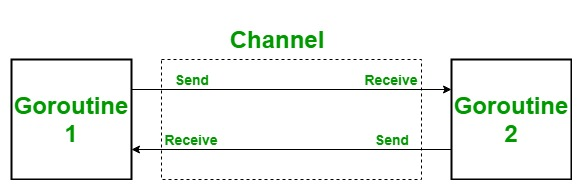In Go language, a channel is created using chan keyword and it can only transfer data of the same type, different types of data are not allowed to transport from the same channel.

Syntax:

`var Channel_name chan Type`

You can also create a channel using make() function using a shorthand declaration.

Syntax:

`channel_name:= make(chan Type)`

The below statement indicates that the data(element) send to the channel(Mychannel) with the help of a <- operator.

`Mychannel <- element`

The below statement indicates that the element receives data from the channel(Mychannel).

`element := <-Mychannel`

If the result of the received statement is not going to use is also a valid statement. You can also write a receive statement as:

`<-Mychannel`

Example:

 `// Go program to illustrate send``// and receive operation``package main`` ` `import ``"fmt"`` ` `func myfunc(ch chan ``int``) {`` ` `    ``fmt.Println(234 + <-ch)``}``func main() {``    ``fmt.Println(``"start Main method"``)``    ``// Creating a channel``    ``ch := make(chan ``int``)`` ` `    ``go myfunc(ch)``    ``ch <- 23``    ``fmt.Println(``"End Main method"``)``}`

Output:

```start Main method
257
End Main method
```

To read more, you can refer to the article Channels in Golang.

### Select Statement

The select statement is just like switch statement, but in the select statement, case statement refers to communication, i.e. sent or receive operation on the channel.

Syntax:

```select{

case SendOrReceive1: // Statement
case SendOrReceive2: // Statement
case SendOrReceive3: // Statement
.......
default: // Statement
```

Example:

 `// Go program to illustrate the``// concept of select statement``package main``  ` `import(``"fmt"`` ``"time"``)``      ` `    ``// function 1``    ``func portal1(channel1 chan string) {`` ` `        ``time``.Sleep(3*``time``.Second)``        ``channel1 <- ``"Welcome to channel 1"``    ``}``      ` `    ``// function 2``     ``func portal2(channel2 chan string) {`` ` `        ``time``.Sleep(9*``time``.Second)``        ``channel2 <- ``"Welcome to channel 2"``    ``}``  ` `// main function``func main(){``      ` `    ``// Creating channels``   ``R1:= make(chan string)``   ``R2:= make(chan string)``     ` `   ``// calling function 1 and ``   ``// function 2 in goroutine``   ``go portal1(R1)``   ``go portal2(R2)`` ` `   ``select{`` ` `        ``// case 1 for portal 1``       ``case` `op1:= <- R1:``       ``fmt.Println(op1)``  ` `       ``// case 2 for portal 2``       ``case` `op2:= <- R2:``       ``fmt.Println(op2)``   ``}``     ` `}`

Output:

`Welcome to channel 1`

Explanation: In the above program, portal 1 sleep for 3 seconds and portal 2 sleep for 9 seconds after their sleep time over they will ready to proceed. Now, select statement waits till their sleep time, when the portal 2 wakes up, it selects case 2 and prints “Welcome to channel 1”. If the portal 1 wakes up before portal 2 then the output is “welcome to channel 2”.

My Personal Notes arrow_drop_up